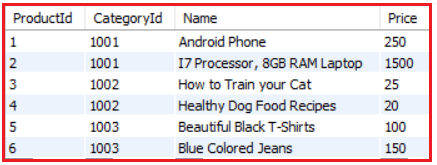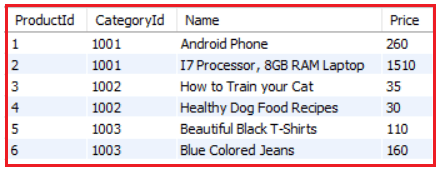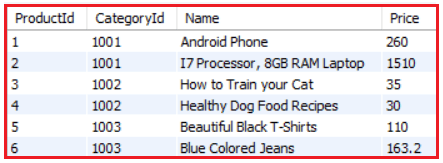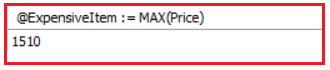# Assignment Operator in MySQL

## Assignment Operator in MySQL with Examples

In this article, I am going to discuss Assignment Operator in MySQL with Examples. Please read our previous article where we discussed SET Operators (UNION, UNION ALL, INTERSECT, & EXCEPT) in MySQL with examples.

##### Assignment Operator in MySQL

The Assignment Operator in MySQL is used to assign or compare a value to a column or a field of a table. The equal sign (=) is the assignment operator where the value on the right is assigned to the value on the left. It is also used to establish a relationship between a column heading and the expression that defines the values for the column.

##### Example to understand Assignment Operator in MySQL

Let us understand the MySQL Assignment Operator with some examples. We are going to use the following Product table to understand the Assignment Operator.Please execute the below SQL Script to create and populate the Product table with the required sample data.

```CREATE DATABASE ShoppingCart;
USE ShoppingCart;

CREATE TABLE Product (
ProductId INT PRIMARY KEY,
CategoryId INT,
Name VARCHAR(100),
Price FLOAT
);

INSERT INTO Product VALUES (1, 1001, 'Android Phone', 250);
INSERT INTO Product VALUES (2, 1001, 'I7 Processor, 8GB RAM Laptop', 1500);
INSERT INTO Product VALUES (3, 1002, 'How to Train your Cat', 25);
INSERT INTO Product VALUES (4, 1002, 'Healthy Dog Food Recipes', 20);
INSERT INTO Product VALUES (5, 1003, 'Beautiful Black T-Shirts', 100);
INSERT INTO Product VALUES (6, 1003, 'Blue Colored Jeans', 150);
```
##### Example: Update the Price of each product by adding 10

Now we will update the Price column of the Product table by using the equals operator as an assignment. Following is the SQL statement.

UPDATE Product SET Price = Price + 10;

Once you execute the above Update statement, now verify that the Price column value in the Product table is updated as shown in the below image. SELECT * FROM Product; will give you the following result set.Here, you can observe the Price column has been updated by raising the existing prices by adding 10. Also, we can use the same operator for comparing values. Following is the example:

UPDATE Product SET Price = Price * 1.02 WHERE ProductId = 6;

Let’s see the updated table: SELECT * FROM Product; will give you the following output.Here we are updating the Price column of the Product table where the ProductId is 6. And you can observe that only the Price with ProductId =6 has been updated.

##### Assigning Variables using Assignment Operator in MySQL

There are two ways to assign a value:

1. By using SET statement: Using SET statement we can either use := or = as an assignment operator.
2. By using SELECT statement: Using SELECT statement we must use := as an assignment operator because = operator is used for comparison in MySQL.
###### Syntax:

SET variableName = expression; where the variable name can be any variable created.
SELECT FieldName = expression; where field name can be any given name.

##### Example: Using SET Statement in MySQL

SET @MyCounter = 1;
SELECT @MyCounter;

In this example, first, we have created a variable @MyCounter and then we are using the assignment operator to set @MyCounter to a value returned by an expression.

##### Example: Using SELECT Statement in MySQL

Let’s get the most expensive item from the Product table and assigns the Price to the variable @ExpensiveItem. Following is the SQL Statement.

SELECT @ExpensiveItem := MAX(Price) FROM Product;

When you execute the above statement, you will get the following output.In the next article, I am going to discussed Constraints in MySQL with Examples. Here, in this article, I try to explain Assignment Operator in MySQL with Examples. I hope you enjoy this article.